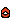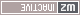My options for improving code speed.

For code related discussions and questions
moltengear
TrainedPosts: 136
Joined: 22 Jul 2017, 15:05

Re: My options for improving code speed.

Cyp wrote:
06 Apr 2019, 20:02
Might be fairer to compare to sqrtf than to sqrt. I'd naïevely guess sqrtf to be faster and more accurate than Q_rsqrt.

I'm not sure square root calculations are the main bottleneck in the game.
Remade! There was a difference of 10 times. Now more than 13 times. So it works!

Cyp
EvitcaniPosts: 742
Joined: 17 Jan 2010, 23:35

Re: My options for improving code speed.

faster ≠ works

Changing slightly, so it could actually compile:

Code: Select all

``````// g++ -O3 -march=native -fno-strict-aliasing -DQ -s -o sqrtf sqrtf.cpp -Wall -Wextra -fno-math-errno

#include <cmath>
#include <iostream>

float Q_rsqrt(float number)
{
int32_t i;
float x2, y;
const float threehalfs = 1.5F;

x2 = number * 0.5F;
y = number;
i = *(int32_t *)&y;
i = 0x5f3759df - (i >> 1);
y = *(float *)&i;
y = y * (threehalfs - (x2 * y * y));

return y;
}

int main() {
float a = 0, t = 0;
#ifdef Q
for (int x = 0; x < 1000000000; x++)
{
a = Q_rsqrt(t);
t = t + 0.01;
float z = Q_rsqrt(a + t);
a = z;
}
#else
for (int x = 0; x < 1000000000; x++)
{
a = 1.f/sqrtf(t);
t = t + 0.01f;
float z = 1.f/sqrtf(a + t);
a = z;
}
#endif
std::cout << a << std::endl;
}
``````
I do actually measure the Q_rsqrt version to be about 13% faster, but the final result doesn't exactly agree (gives 0.00194982 instead of 0.00195312). Even if it was infinitely faster, I don't think it would affect the game performance significantly.

——————————————

Note that without -fno-math-errno, the sqrtf version is about 133% slower. The game only uses errno in one place, so should probably be compiled with -fno-math-errno if that can be removed.